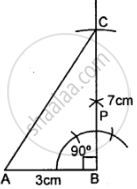# Construct Traingle Abc, When : Ab = 3 Cm, Bc = 7 Cm and ∠B = 90°. - Mathematics

Diagram
Sum

Construct traingle ABC, when : AB = 3 cm, BC = 7 cm and ∠B = 90°.

#### Solution

Steps of Construction:

(1) Draw a line segment AB = 3 cm(2) With the help of compasses, construct ∠ABC = 90°.

(3) With B as centre, draw an arc of 7 cm length which cuts BP at C.

(4) Join A and C.

(5) Triangle ABC, so obtained, is the required triangle.

Concept: Classification of Triangles (On the Basis of Sides, and of Angles)
Is there an error in this question or solution?

#### APPEARS IN

Selina Class 6 Mathematics
Chapter 26 Triangles (Including Types, Properties and Constructions)
Exercise 26 (B) | Q 5